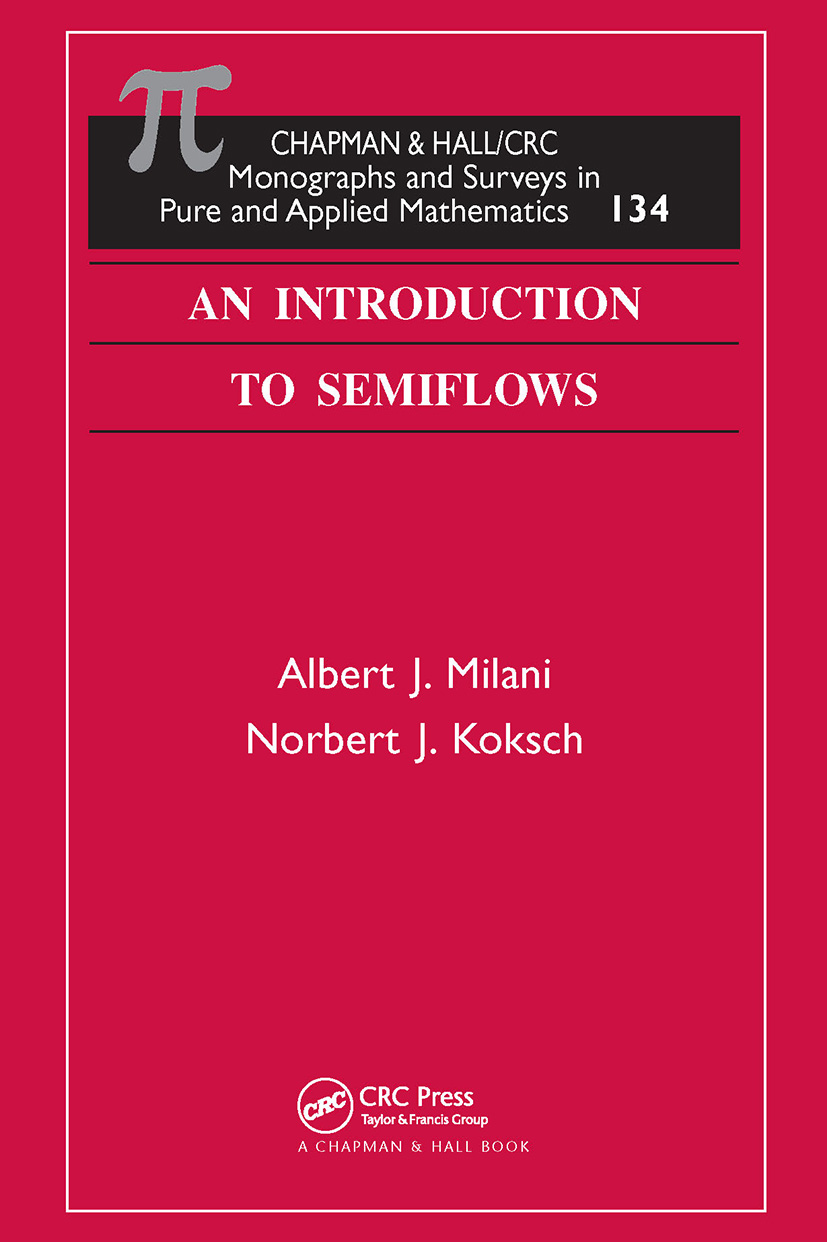# An Introduction to Semiflows

## 1st Edition

Chapman and Hall/CRC

386 pages | 26 B/W Illus.

Hardback: 9781584884583
pub: 2004-10-14
\$170.00
x
eBook (VitalSource) : 9780429147111
pub: 2004-10-14
from \$28.98

FREE Standard Shipping!

### Description

This book introduces the class of dynamical systems called semiflows, which includes systems defined or modeled by certain types of differential evolution equations (DEEs). It focuses on the basic results of the theory of dynamical systems that can be extended naturally and applied to study the asymptotic behavior of the solutions of DEEs. The authors concentrate on three types of absorbing sets: attractors, exponential attractors, and inertial manifolds. They present the fundamental properties of these sets, and then proceed to show the existence of some of these sets for a number of dynamical systems generated by well-known physical models. In particular, they consider in full detail two particular PDEEs: a semilinear version of the heat equation and a corresponding version of the dissipative wave equation. These examples illustrate the most important features of the theory of semiflows and provide a sort of template that can be applied to the analysis of other models.

The material builds in a careful, gradual progression, developing the background needed by newcomers to the field, and culminating in a more detailed presentation of the main topics than found in most sources. The authors' approach to and treatment of the subject builds the foundation for more advanced references and research on global attractors, exponential attractors, and inertial manifolds.

### Reviews

"I am convinced that the authors have made this mathematically-demanding area as accessible as possible to the newcomer in the field. The pictures and 'simple ODE applications' help the reader gain intuition. This book does a fine job of presenting difficult material on applications to readers without in-depth knowledge of partial differential equations in a painless and enjoyable way."

- Hal Smith, Arizona State University

DYNAMICAL PROCESSES

Introduction

Ordinary Differential Equations

Attracting Sets

Iterated Sequences

Lorenz' Equations

Duffing's Equation

Summary

ATTRACTORS OF SEMIFLOWS

Distance and Semidistance

Discrete and Continuous Semiflows

Invariant Sets

Attractors

Dissipativity

Absorbing Sets and Attractors

Attractors via a-Contractions

Fractal Dimension

A Priori Estimates

ATTRACTORS FOR SEMILINEAR EVOLUTION EQUATIONS

PDEEs as Dynamical Systems

Functional Framework

The Parabolic Problem

The Hyperbolic Problem

Regularity

Upper Semicontinuity of the Global Attractors

EXPONENTIAL ATTRACTORS

Introduction

The Discrete Squeezing Property

The Parabolic Problem

The Hyperbolic Problem

Proof of Theorem 4.4

Concluding Remarks

INERTIAL MANIFOLDS

Introduction

Definitions and Comparisons

Geometric Assumptions on the Semiflow

Strong Squeezing Property and Inertial Manifolds

A Modification

Inertial Manifolds for Evolution Equations

Applications

Semilinear Evolution Equations in One Space Dimension

EXAMPLES

Cahn-Hilliard Equations

Beam and von Kármán Equation

Navier-Stokes Equations

Maxwell's Equations

A NON-EXISTENCE RESULT FOR INERTIAL MANIFOLDS

The Initial-Boundary Value Problem

Overview of the Argument

The Linearized Problem

Inertial Manifolds for the Linearized Problem

C1 Linearization Equivalence

Perturbations of the Nonlinear Flow

Asymptotic Properties of the Perturbed Flow

The Non-Existence Result

Proof of Proposition 7.17

The C1 Linearization Equivalence Theorems.

APPENDIX: SELECTED RESULTS FROM ANALYSIS

A.1 Ordinary Differential Equations

A.2 Linear Spaces and their Duals

A.3 Semigroups of Linear Operators

A.4 Lebesgue Spaces

A.5 Sobolev Spaces of Scalar Valued Functions

A.6 Sobolev Spaces of Vector Valued Functions

A.7 The Spaces H(div,W) and H(curl,W)

A.8 Almost Periodic Functions

BIBLIOGRAPHY

INDEX

NOMENCLATURE

Albert J. Milani is a professor in the Department of Mathematics, University of Wisconsin-Milwaukee, USA.

Norbert J. Koksch is a docent in the Department of Mathematics, Technische Universität, Dresden, Germany